Search
• Annabelle Dempsey

## The History, Ecology, and Geology of Virgin Falls State Natural Area

Updated: Mar 8

• Annabelle Dempsey

## Susceptibility of Cookeville, Tennessee to Runoff based on a Three-Hour Rainfall Event

Updated: May 5, 2020

Susceptibility of Cookeville, Tennessee to Runoff based on a Three-Hour Rainfall Event was calculated taking into account impervious surfaces, precipitation data, and soil survey data.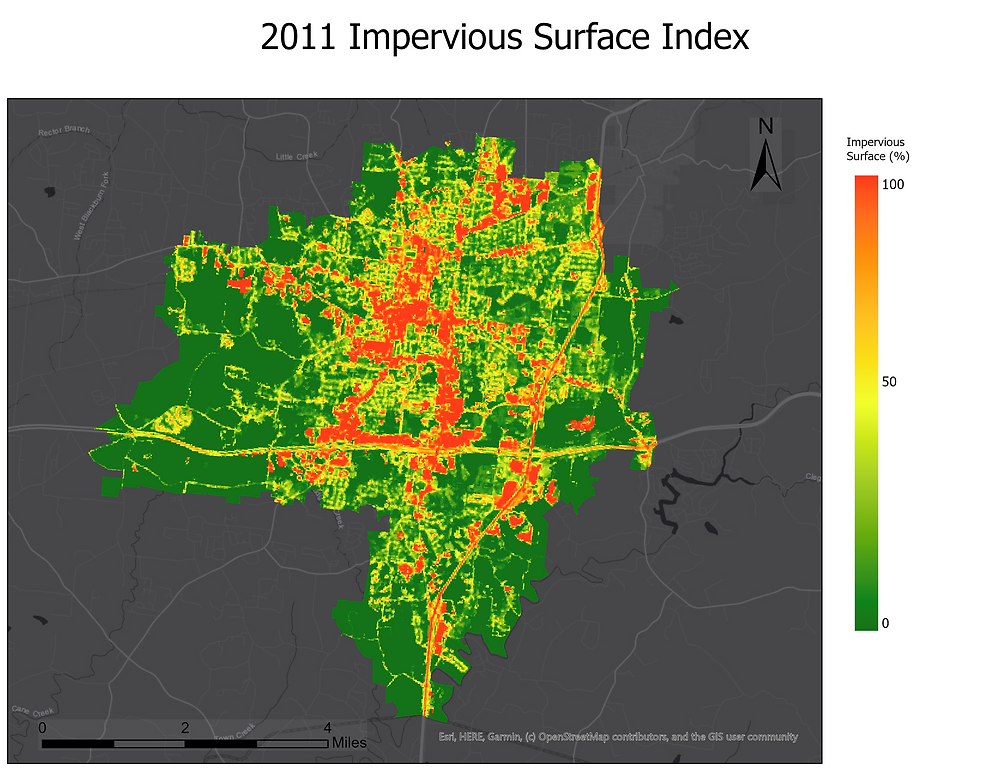In order to calculate potential flooding, a runoff model must first be run. The model is expressed as the following formula (2);

`R = P - F  `

Where:

``` R = Runoff
P = Cumulative Rainfall expressed as cubic feet
F = Cumulative Infiltration ```

The study upon which this methodology is based uses a modified form of the Runoff Model, expressed as the following formula;

`R = P - q `

Where;

```R = Runoff
P = Cumulative Rainfall expressed as cubic feet```

Calculation of q

An adjustment Index was first calculated for each impervious surface layer using a modified Green-Ampt Model, as per Lindberg, Joakim (2).This formula estimates the influence of landuse on infiltration by applying an adjustment index to impervious surfaces, based on the percentage of impervious surface area in each cell of the study area.

This new impervious surface raster is calculated using the following formula;

Green-Ampt Model

`Q = 1 - U`

Where:

```Q = Impervious Surface Adjustment Index
U =  percentage impervious surface```

This was accomplished through the following workflow;

1. Use Create Random Raster

• Extent and resolution must be the same as the Impervious Surface rasters

• Cell size = 30

• Raster type = 8 bit unsigned

• Band number = 1

• Distribution = uniform

• The minimum value is set to 1

• The maximum value is set to 1

• Output coordinate system: Albers Conical Equal Area

Run the tool to create RasterValue1

2. Use Raster Calculator

• Impervious Surface - RasterValue1

The result is the Impervious Surface Adjustment Index (Q) for each Impervious Surface Raster used in calculations (for the purpose of this report, years 2006, 2011, and 2016).The variable F was estimated based upon Web Soil Survey data and the aid of Table 1, Morgan (2005).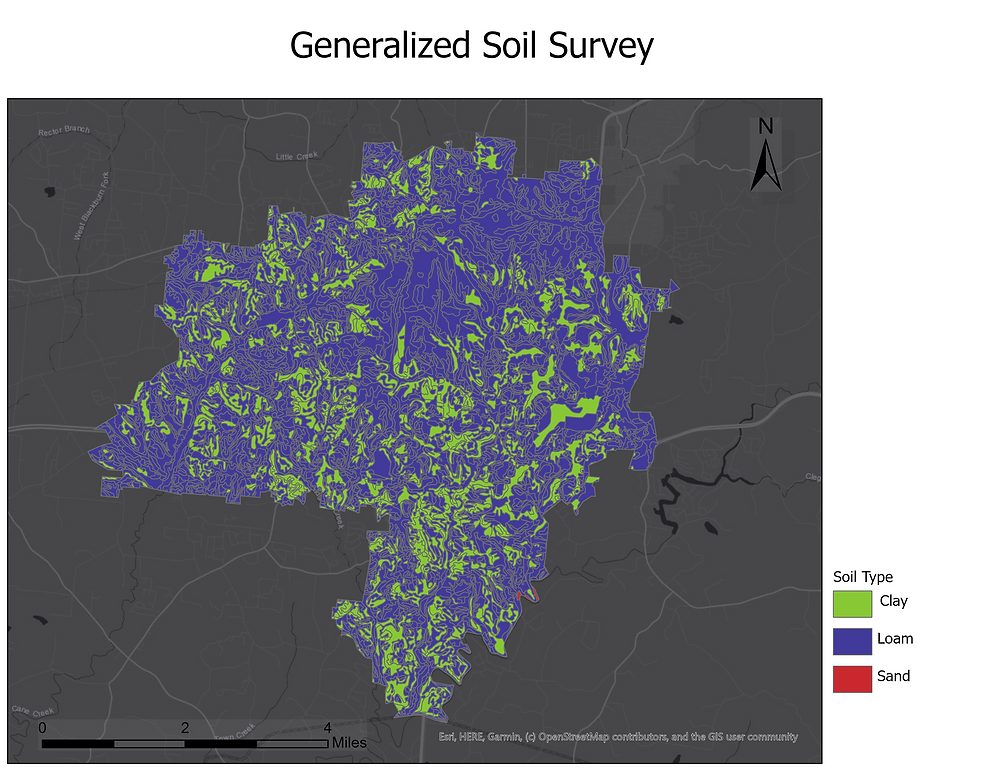Figure 1: Estimated infiltration rates for three different soils. The graph is adapted after Morgan (2005)

Clay was estimated to have an infiltration rate of 6.25 mm/h (20mm/h /3.20t).

Loam was estimated to have an infiltration rate of 10.94 mm/h (35mm/h /3.20t).

Sand was estimated to have an infiltration rate of 17.19 mm/h (55 mm/h /3.20t).

These values were then appended to their respective soil groups in the soil survey layer.

Polygon to Raster was then ran on this layer and the resulting raster acted as the variable F.Raster Calcuator was then used to calculate Adjusted Infiltration for each Impervious Surface raster through the following formula;

```q = F * Q

```

Where;

```q = Adjusted Infiltration
F = Cumulative Infiltration
Q = Impervious Surface Adjustment Index

```

The expression used to accomplish this was F * ImperviousSurfaceAdjustmentIndex.

The end results, q2006, q2011, and q2016 were then set aside until after P had been calculated.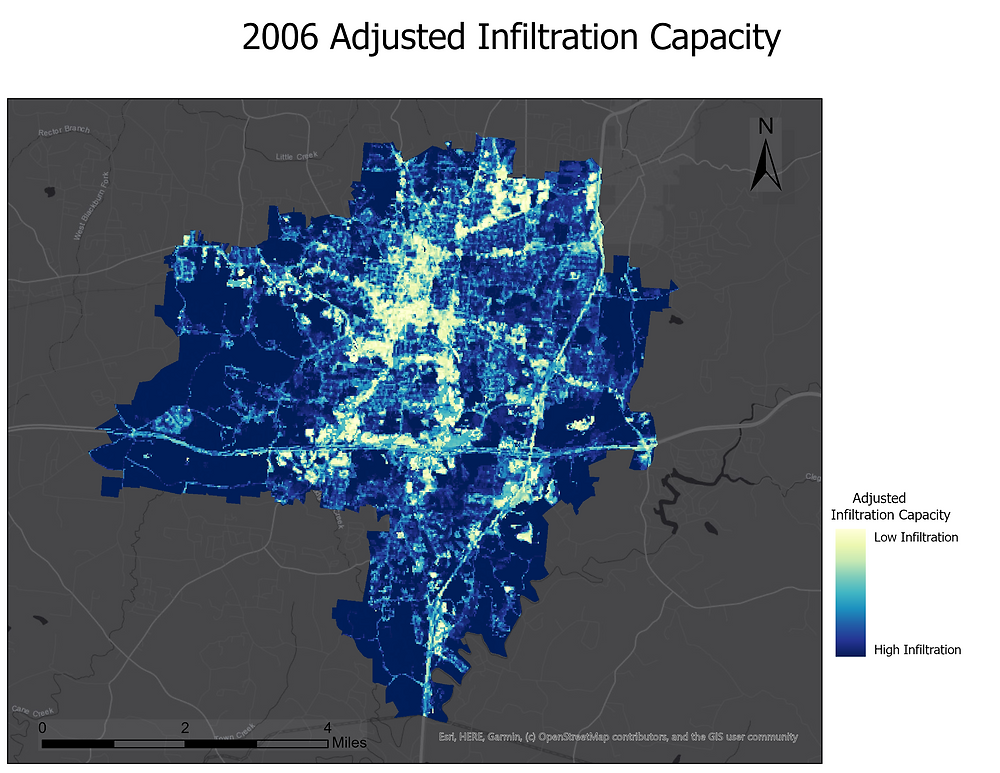Calculation of P

P or Cumulative Rainfall, per the study referenced, was based off a three-hour rainfall event for the study area. Data to determine this was collected from The National Oceanic and Atmospheric Administration using their Advanced Hydrological Prediction data portal, The data the study referenced used fifteen-minute intervals which were not available for Cookeville, TN. Data from the closest existing station in Monterey, TN was substituted. This date ranged from 1/1/2006-1/1/2014, the closest approximation to the ten year study period as covered by the impervious surface layers.

The mean and max (shown below histogram) were calculated for the dataset to find a suitable three-hour rainfall event.The max for the dataset was 2.52/QGAG (precipitation expressed as inches).

This max value resides in the portion of the dataset that took place on 2011-11-28 during the hours of 12:30 AM and 10:00 PM.

P was then calculated using this time range until the three hour mark was researched. The results can be seen in Table 2;Table 2: Cumulative Rainfall for three-hour event occurring on 2011-11-28

Red denotes the time, precipitation level, and cumulative rain in inches as they were when the three-hour mark was reached. However, due to the sampling times within this subset, the actual event time was 3.15 hours.

F, 9.3, was then appended to a new raster using the following workflow;

1. Use Create Random Raster

• Extent and resolution must be the same as the Impervious Surface rasters

• Cell size = 30

• Raster type = 8 bit unsigned

• Band number = 1

• Distribution = uniform

• The minimum value is set to 9.3

• The maximum value is set to 9.3

• Output coordinate system: Albers Conical Equal Area

Run the tool to create P_3_Hour_Event .

Proceed, using P and q, to calculate the Modified Runoff Formula

Modified Runoff Formula

`R = P - q `

Where;

```R = Runoff
P = Cumulative Rainfall expressed as cubic feet```

Use Raster Calculator to subtract q(Year) from P_3_Hour_Event for each impervious surface.

The end result of these steps is the following maps which show areas at risk of forming runoff during a three-hour event similar to the one that occurred on 2011-11-28.-------------------------------------------------------------------------------------------------- References

A GIS-based model for urban flood inundation - ScienceDirect. (n.d.). https://www.sciencedirect.com/science/article/pii/S0022169409002546

Lindberg, Joakim. "Locating potential flood areas in an urban environment using remote sensing and GIS, case study Lund, Sweden."Student thesis series INES(2015).

National Centers for Environmental Information, N. (n.d.). Monterey, Tennessee Precipitation 15 Minute Station Details.

NCLD Urban Imperviousness 2006, 2011, 2016. (n.d.).

• Annabelle Dempsey

## Upper Cumberland Illegal Dumpsites Predictive Model Refined (Technical Report/Procedures)

Updated: Apr 21, 2020

Without County Overlay:With County Overlay:___________________________________________________________________________________ Procedures

The following procedures were adapted from Viazanko, Andrea, "Predictive Model of Illegal Dumpsites in Westmoreland and York Counties, Pennsylvania" (2017). Theses and Dissertations (All). 1561. The sample size for the Illegal Dumpsite dataset is 46 .

Road shapefiles were obtained from TDOT.

Euclidean Distance was used upon the roads polylines.

Extract by Mask was used to cut the extent down to the Upper Cumberland Region.

Road/Euclidean Distance values were extracted to UC Illegal Dumpsites point layer.

The following bar chart was created from this layer;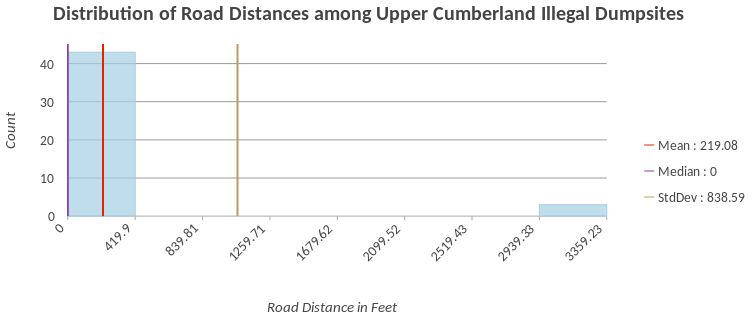This chart yielded the following value ranges, ranked from highest to lowest occurrence among dumpsites:

• 0-419.00 with 43 occurrences

• 2939.3-3359.3 with 3 occurrences

These value ranges were reclassed as follows:

• 0-419.00 = 1

• 2939.3-3359.3 = 2

Raster surface was then set aside until final step.

Landcover:

NCLD 2016 Landcover data was used.

Extract by Mask was used to cut the study area down to the Upper Cumberland Region.

The NCLD dataset was then extracted to the UC Illegal Dumpsites Point Layer.

Landuse was then summarized by count and the following bar chart produced;It was determined, using this chart, that the following land uses occurred most often;

• Developed, Open Space with 32 occurrences.

• Deciduous Forest with 5 occurrences.

• Developed, Low Intensity and Hay/Pasture with 3 occurrences per category.

• Developed, Medium Intensity and Mixed Forest with 1 occurrence per category

The NCLD raster surface was then reclassified using the following values (Method 1);

• Developed, Open Space = 1

• All other categories = 2

The NCLD raster surface was also reclassified using a different set of values, as follows (Method 2);

• Developed, Open Space and Deciduous Forest = 1

• Developed, Low Intensity and Hay/Pasture = 2

• All other categories = 3

These raster surfaces were then set aside until the final steps.

Population:

2017 Block Group polygon data from the Census Burea (obtained with the help of Chuck Sutherland) was used.

Calculate Geometry was used to calculate area, expressed as square kilometers, for each block group.

Calculate field was then used to calculate population density per square kilometer for each block group. Formula was as follows;

Population (B01001m1)/Area = Population Density per Square Kilometer

Polygon to Raster was then used upon the blockgroup.

Values from this raster were then extracted to UC Illegal Dumpsites layer. The following bar chart was created using this data;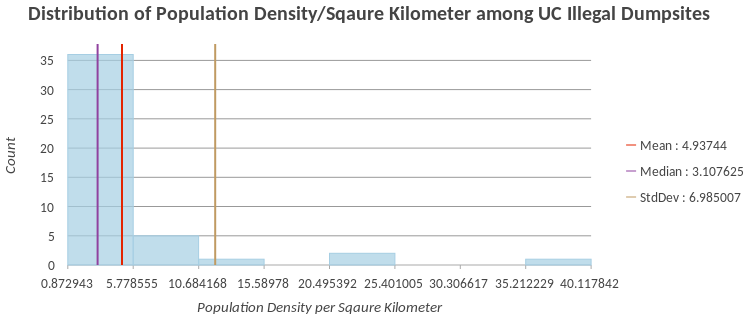The most common value ranges from this chart are as follows;

• 0.87-5.7 with 36 occurrences

• 5.8-10.6 with 5 occurrences

• 20.4-25.4 with 2 occurrences

• 10.7-15.5 with 1 occurrence

• 35.2-40.1 with 1 occurrence

The raster surface was then reclassified as such;

Pop Density/sqr kilom

• 0-5.7 = 1

• All other values (5.8-40.1) = 2

Raster surface was then set aside until final steps.

Slope:

TNDEM (obtained from Chuck Sutherland)

Extract by mask was used to cut the study area down to the Upper Cumberland Region.

Slope was ran on the resulting file using percent rise rather than degree.

Slope values were then extracted to UC Illegal Dumpsites Point Layer. The following bar chart was created from this data;The chart showed the following ranges were typically found within dumpsites, ranked most to least;

• 0.74-5.4 with 13 occurrences

• 5.5-10.1 with 11 occurrences

• 10.2-14.8 with 6 occurrences

• 14.9-19.6 with 5 occurrences

• 19.7-24.3 with 3 occurrences

• 24.4-29.0 with 2 occurrences

The raster surface was then reclassified with the following values;

• 0-10.1 = 1

• 10.2-19.6 and 29.1-33.7 = 2

• 19.7-29.0 and 33.8-38.4 = 3

Raster surface was then set aside until final steps

Final Steps

Weighted Sum was used to combine the above raster files using the following weights;

• Population = 0.23

• Slope = 0.15

• Land Use = 0.15

The productive surface was then symbolized based upon a stretch type of equalized histogram.

Two models were produced from this process. Only the first, which used the Land Use reclassifcation found in Method 1, is shown as there is no discernabke difference between models made with Method 1 Land Use data and Method 2 Land Use data. _______________________________________________________________________________________

I conclude that this model needs further refinement due to the following limitations; A sample size of 46 dumpsites may be too small to accurately create reclassify variables for use within a predictive surface.

Reclassification scheme may need to be tweaked once further statistical analysis of each variable is conducted.